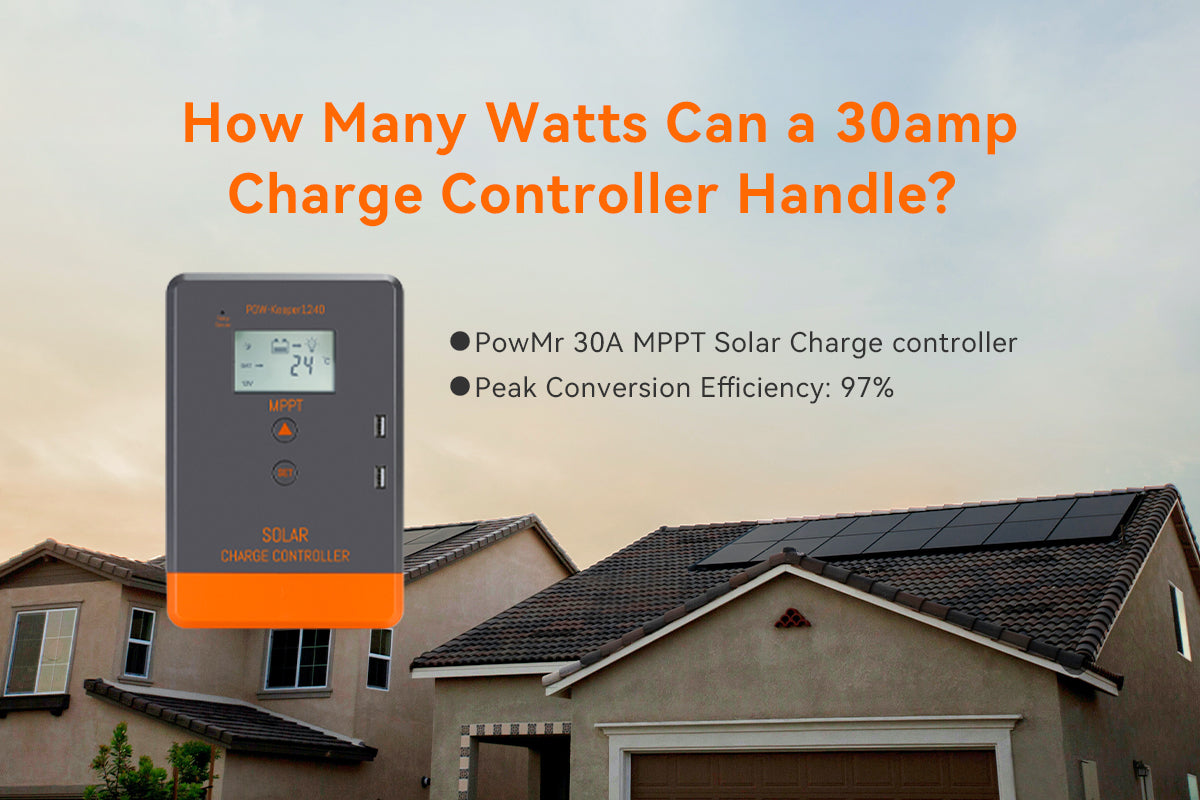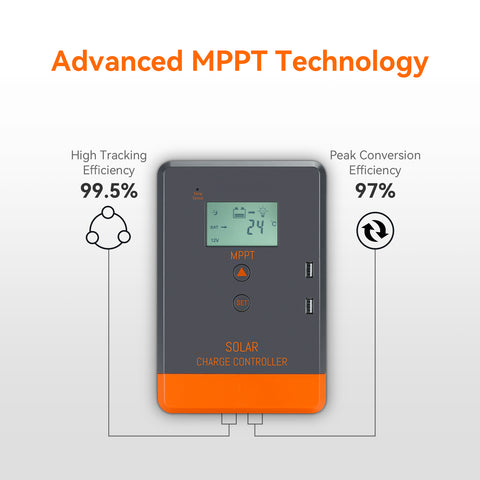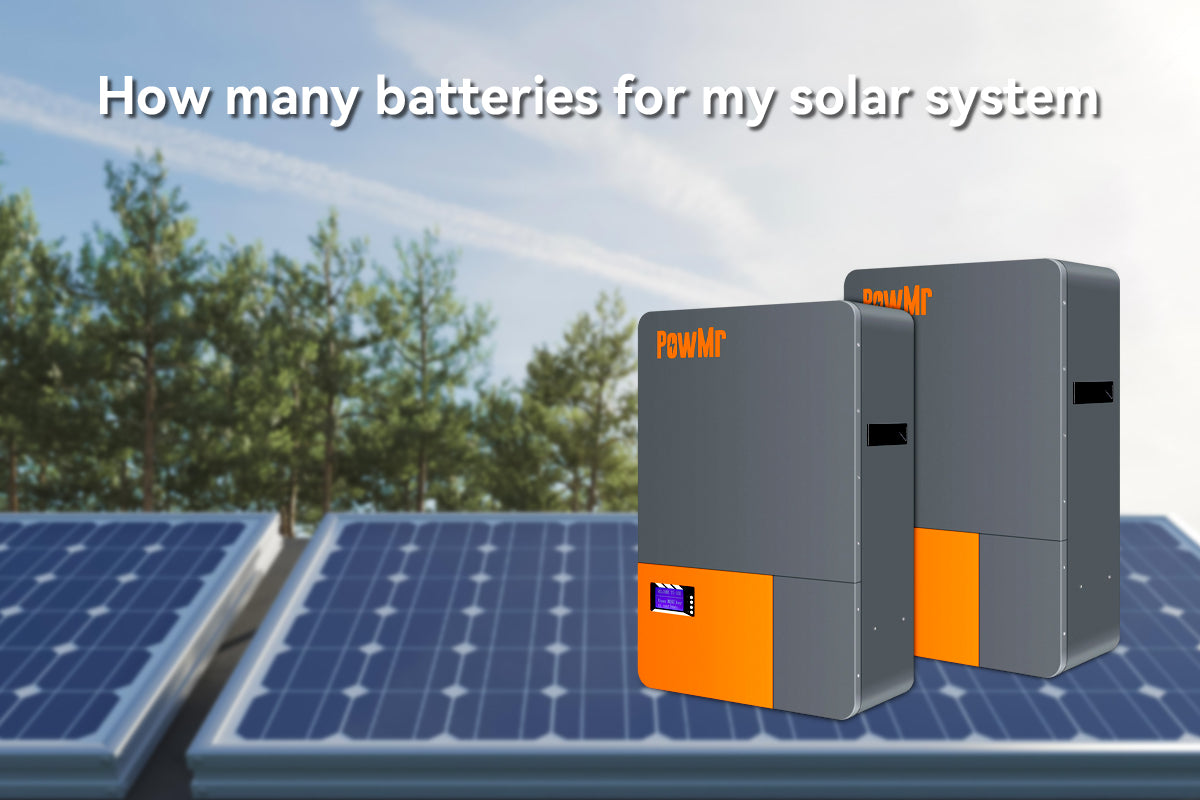# How Many Watts Can a 30 amp Charge Controller Handle?When setting up a solar power system, it's crucial to understand the capabilities of the various components involved. One such component is the solar charge controller, which plays a vital role in regulating the flow of energy from the solar panels to the battery bank.

If the current question is: How many watts can 30amp handle?
You might say: "Amperage x Voltage".

Yes, the question of how many watts are in 30amp can indeed be calculated by this formula:
You might say: "Amperage x Voltage".

Assuming a system voltage of 120, then a 30-amp circuit can handle up to 3600 watts of power.

In fact, it is not that easy for us when dealing with the solar charge controllers that can have more than one input voltage and output voltage.

In this post, we will explore the power limits of a 30amp solar charge controller and shed light on how many watts it can handle and the two influencing factors can be easily overlooked.

## Two influencing factors of how many watt can a30amp solar charge controller handle

### Factor 1 - The Conversion Efficiency of the Solar Charge Controller

The conversion efficiency of a solar charge controller refers to how effectively it can convert the solar power received from the solar panels into usable electricity for charging the batteries. A higher conversion efficiency means that more of the available solar power is effectively utilized, while less is lost as heat or in the conversion process.

When it comes to conversion efficiency, it is easy to associate the difference between pwm and mppt solar controllers, as there are differences in their conversion efficiency and the resulting handling of wattage.

• PWM Solar Charge Controller
PWM charge controller operates by rapidly switching the solar panel's current on and off to maintain the battery voltage. PWM charge controllers are generally less efficient compared to MPPT controllers. They typically have a conversion efficiency ranging from 70% to 90%.

• MPPT Solar Charge Controller
An MPPT charge controller employs advanced algorithms to track the maximum power point of the solar panel array, optimizing power conversion. They typically have a conversion efficiency ranging from 90% to 99%.

For example, POW-KEEPER1230 ensures optimal utilization of solar energy achieving a remarkable maximum conversion efficiency of up to 97% with high-quality components to enhance system performance.Therefore, how many watts a 30amp solar charge controller can handle, the conversion efficiency becomes a crucial factor. The maximum wattage capacity of a 30amp charge controller is determined by its current rating, which remains the same for both PWM and MPPT charge controllers.

### Factor 2 - System Voltage

The system voltage in a solar power system comprises two key components: the battery voltage and the solar panel voltage. Amoung them, the battery voltage has an direct impact on the maximum wattage capacity of a 30amp solar charge controller.

The battery voltage refers to the voltage at which the solar batteries are connected in the system. Common battery voltages for solar power systems include 12 volts24 volts, and 48 volts. The battery voltage affects the maximum wattage capacity of the charge controller because it determines the amount of power that can be transmitted at a given amperage.

Note:
Although the voltage of solar panels does not directly affect the maximum wattage that a solar charge controller can handle, it influences the power transmission capabilities. So, when selecting a solar charge controller, it is essential to ensure that its specifications match the solar panel voltage.

## Calculation - How Many Watts Can a Solar Charge Controller Handle

Now that we have understood the factors that affect how many watts a solar charge controller can handle, we can put that knowledge into practice and perform the calculations.

According to the above explanation, it is not difficult to derive the formula for how many watt can a solar charge controller handle:

• Wattage = Amperage x Voltage x Conversion Efficiency of the Solar Charge Controller

Assuming the solar system operates at a 12volt/24volt battery voltage, we take a MPPT solar charge controller with a peak conversion efficiency of 97% as an example to calculate:

### How many watts can a 30amp solar charge controller handle

In this scenario, a 30amps solar charge controller connected to a 12volt battery system can handle a maximum of 349.2 watts of power:

Wattage = Amperage x Voltage x Conversion Efficiency of the Solar Charge Controller
= 30Amps x 12V x 0.97 = 349.2Watts

If charging a 24 volt battery bank at 30 amps, then the power calculation is:
30 amps x 24 volts x 0.97= 698.4 watts

### How Many Watts Can a 60 amp Charge Controller Handle?

60A Charge controller watts depends on the output charging voltage. For a 12 volt charging system, the power in watts would be:

60 amps x 12 volts x 0.97 = 700.8 watts

If charging a 24 volt battery at 60 amp, then the power calculation is:

60 amps x 24 volts x 0.97 = 1441.6 watts

MPPT controllers are often able to handle more power, as they operate in a completely different way.

### How Many Watts Can a 50 amp Charge Controller Handle?

If charging at 50 amps for a 12 volt solar battery, it would be 50 amps x 12 volts x 0.97 = 582 watts

If charging at 50 amps for a 24 volt solar battery, it would be 50 amps x 24 volts x 0.97 = 1164 watts

### How Many Watts Can a 100 amp Charge Controller Handle?

As the current rating of solar chargers get higher, the output charging voltage increases, particularly with MPPT technology.

A good quality MPPT charge controller may have output settings of 12, 24, 36 and 48 volts. In each case the power being handled would be different:

If charging at 100 amps for a 12 volt solar battery, it would be 100 amps x 12 volts x 0.97 = 1164 watts

If charging at 100 amps for a 24 volt solar battery, it would be 100 amps x 24 volts x 0.97 = 2328 watts

If charging at 100 amps for a 36 volt solar battery, it would be 100 amps x 36 volts x 0.97 = 3492 watts

If charging at 100 amps for a 48 volt solar battery, it would be 100 amps x 48 volts x 0.97 = 4656 watts

### How Many Watts Can a 20 amp Charge Controller Handle?

Lower current devices generally specify one PV voltage input of 12 volt nominal (22V Voc) and one output charge voltage of 12V.

20 amps x 12 volts x 0.97 = 233.6 watts

### How Many Watts Can a 40 amp Charge Controller Handle?

MPPT or PWM 40 amp charge controllers may or may not have variable input PV and battery charging output volts, but let’s assume 2 possible output voltages of 12 and 24 volts:

For 12v battery: 40 amps x 12 volts x 0.97 = 465.6 watts

For 24v battery: 40 amps x 24 volts x 0.97 = 931.2 watts

Note: These revised calculations take into account the multiplication by the conversion efficiency factor of 0.97 to provide a more accurate estimation of the wattage that each charge controller can handle.

## Conclusion

While understanding the power limits is essential, it's crucial to consider a few additional factors:

• Voltage Compatibility
Different charge controllers are designed for specific voltage systems (12V, 24V, 48V, etc.). Ensure that the charge controller's voltage rating aligns with your system requirements.

• Efficiency and Losses
It's important to note that the power rating of the solar charge controller refers to the maximum power it can handle under ideal conditions. Real-world factors like temperature, wiring losses, and system inefficiencies may slightly affect the overall performance.

It is recommended to select a charge controller with a capacity slightly higher than the maximum power output of the solar panels. This allows for system expansion and ensures optimal performance.

Note:
The power handling capacity may vary depending on the specific model and manufacturer of the charge controller. Always refer to the manufacturer's specifications and guidelines for accurate information.